# 小丞同学

Author

📢 大家好，我是小丞同学，一名大二的前端爱好者

📢 这篇文章将讲解数据结构中的图

📢 非常感谢你的阅读，不对的地方欢迎指正 🙏

📢 愿你忠于自己，热爱生活

• 什么是图结构？
• 图结构有什么应用场景？
• 图结构有什么方法？
• 如何实现一个图结构？
• LeetCode 实战

📢 碎碎念

## 一、什么是图结构？ #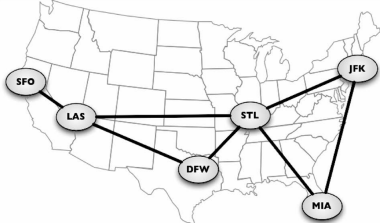• 因为图中的每一条边都是由两个节点相连而成的，因此图可以表示任何二元关系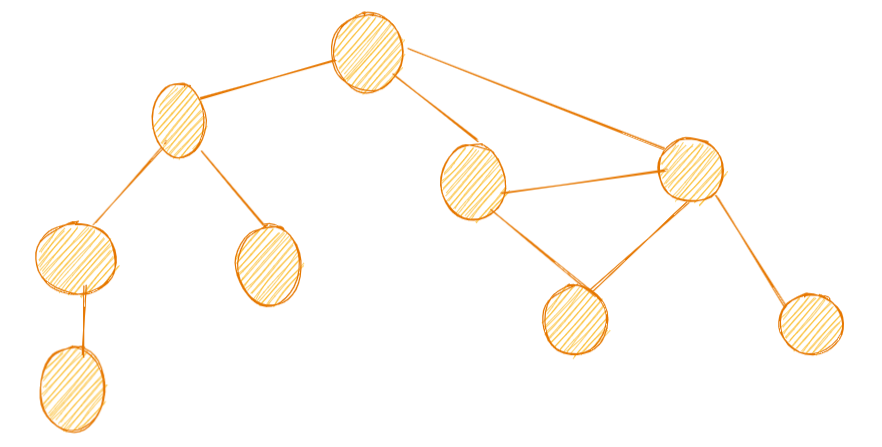`JS` 中没有图结构，我们可以用对象或者数组来构建一个图结构

• 邻接矩阵
• 邻接表
• 关联矩阵

## 二、图的相关术语 #

``````const graph = {
0: [1, 2],
1: ,
2: [0, 3],
3: 
};
``````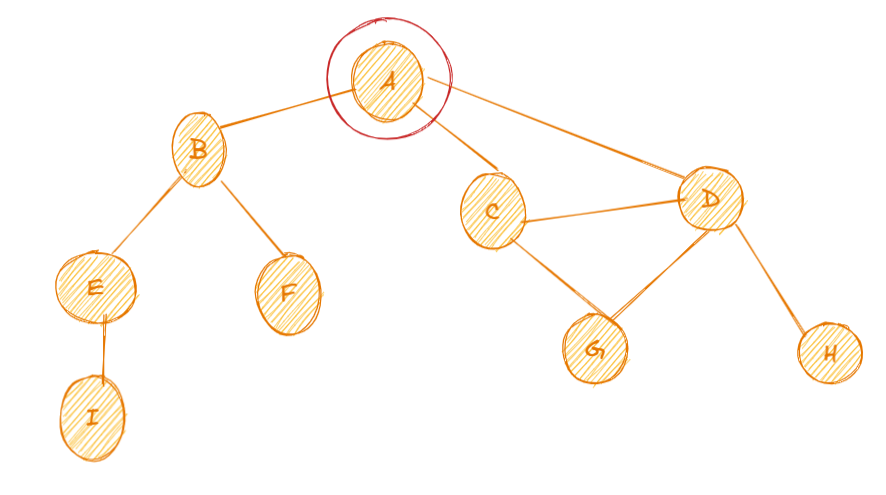• `A`节点和 `B` 节点相邻，`A``D` 是相邻的，`A``C` 是相邻的，`A``E` 不是相邻的，因此 `A` 节点和 `B,C,D` 是相邻节点
• 图中的每一个节点都能作为顶点存在
• `A` 节点的度，由于 `A` 与其他三个节点相连，因此 `A` 节点的度为 `3` ，图中的 `D` 节点和其他 `4` 个节点相连，因此它的度为 `4`
• 可以看到图中 `CDG` 形成了一个环，因此这个图也称为有环的
• 如果图中每两个顶点间存在路径，则图是连通的

### 有向图 #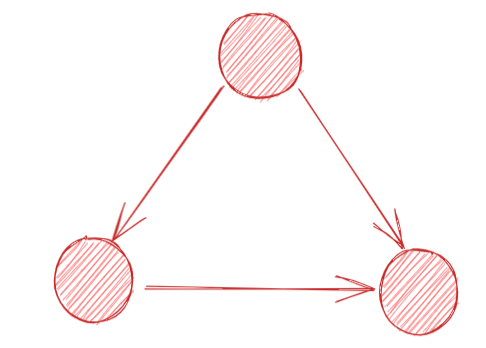### 无向图 #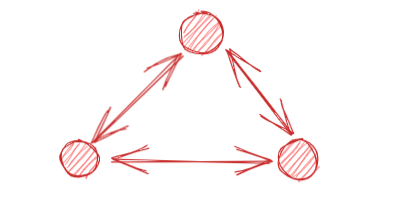## 三、如何表示一个图？ #

### 1. 邻接矩阵 #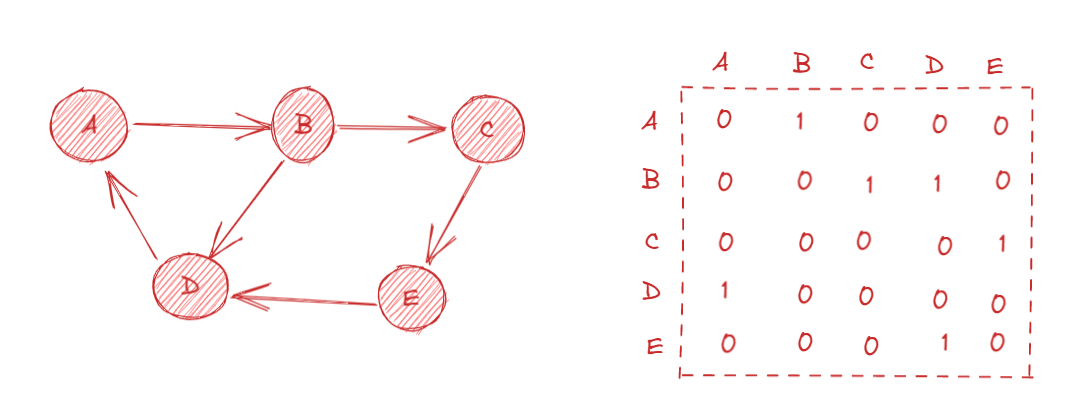### 2. 邻接表 #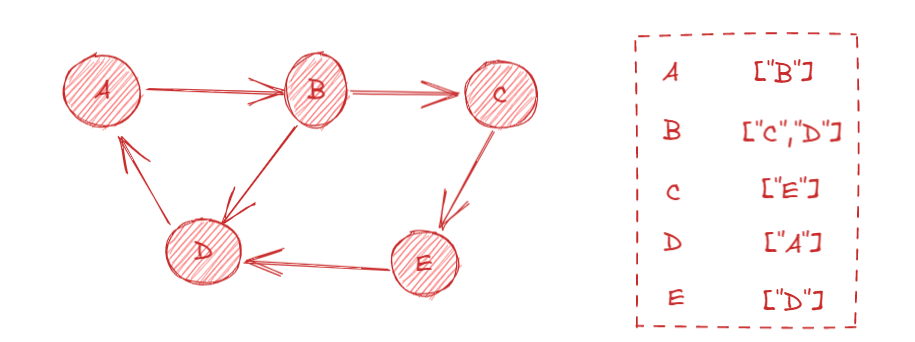## 四、图的操作 #

``````const graph = {
0:[1, 2],
1:,
2:[0, 3],
3:
}
``````

### 1. 深度优先遍历（DFS） #

• 先访问根节点
• 对根节点的没访问过的相邻节点挨个进行深度优先遍历

``````// 记录访问过的节点
const visited = new Set()
// 深度优先遍历
const dfs = (n) => {
console.log(n);
// 获取所有相邻节点
graph[n].forEach(c => {
// 如果没有访问过
if (!visited.has(c)) {
dfs(c)
}
})
}
// 根节点
dfs(2)
``````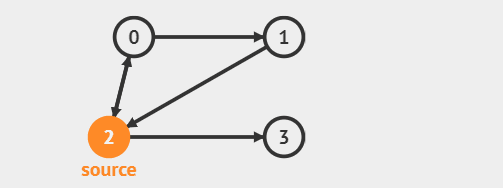### 2. 广度优先遍历（BFS） #

1. 新建一个队列，把根节点入队并访问
2. 把对头没有访问过的相邻节点入队
3. 重复，直至队列为空

``````// 广度优先遍历
const bfs = (n) => {
const visited = new Set();
const q = [n];
while (q.length) {
const n = q.shift();
console.log(n);
graph[n].forEach(c => {
if (!visited.has(c)) {
q.push(c)
}
});
}
}
``````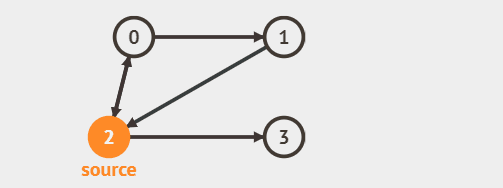## 五、图结构有哪些方法？ #

`addVertex(value)`向图中添加一个顶点
`addEdge(a,b)`向图中添加两点之间的边
`getVertices()`返回图的顶点列表
`toString()`以字符串的形式输出

## 六、手写实现无向图结构 #

### 1. 创建 Graph 类 #

``````class Graph {
constructor() {
this.vertices = [] // 保存顶点
this.edges = [] // 保存边，相当于邻接表
}
}
``````

### 2. 实现 addVertex 方法 #

``````addVertex(value) {
// 如果没有这个顶点
if(!this.vertices.includes(value)){
this.vertices.push(value) // 添加到顶点列表中
this.edges[value] = []    // 添加到邻接表中
}
}
``````

### 3. 实现 addEdge 方法 #

``````addEdge(a,b) {
if(this.vertices.includes(a) && this.vertices.includes(b)) {
if(!this.edges[a].includes(b)) {
this.edges[a].push(b)
this.edges[b].push(a)
}
}
}
``````

### 4. 实现 getVertices 方法 #

``````getVertices() {
return this.vertices
}
``````

### 5. 实现 toString 方法 #

• 先遍历顶点列表
• 在邻接表中找到顶点列表对应的对象
• 拼接字符串，实现输出
``````toString() {
let s = "";
// 遍历图的顶点列表
for (let i = 0; i < this.vertices.length; i++) {
// 采用模板字符串进行拼接
s += `\${this.vertices[i]} -> `;
// 获取顶点对应的邻接表数组
const neighbors = this.edges[this.vertices[i]]
//遍历该邻接表数组，解构数组成字符串
for (let j = 0; j < neighbors.length; j++) {
s += `\${neighbors[j]} `;
}
// 每一个顶点单独成行
s += "\n";
}
return s;
}
``````

### 6. 演示 #

``````const graph = new Graph()
console.log(graph.toString());
``````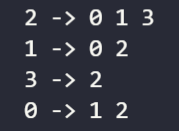## 🐣 彩蛋 #

💌 如果文章有什么错误的地方，或者有什么疑问，欢迎留言，也欢迎私信交流